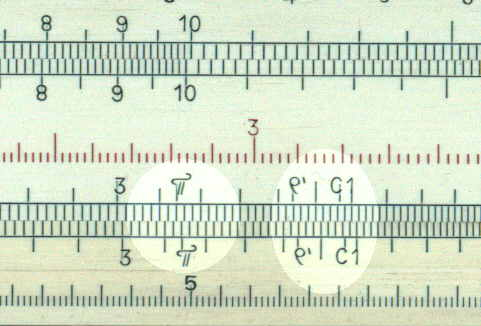HOME INSTRUCTIONS A-to-Z

Gauge points

The are certain numbers which are used frequently in calculation; an obvious example is p (3.142). To save the user having to remember these values they are sometimes marked on the rule with a symbol to indicate their purpose. The following table gives some of the commonest gauge points, the normal and alternative designation and the value. The value is given twice, the "nominal value" is where you would find it on the rule and the "true value" is the value used for calculations.

 Normal Designation Alternative Designation Nominal Value True Value Uses c 1.13 1.128 =Ö (4/p) - volume of a cylinder 1.146 1146 (180 x 60)/(p x 3) the "Gunner's mark" (See below for more details.) r" s 2.06 20655 seconds per radian p 3.142 3.142 r' m 3.44 3438 minutes per radian c1 C 3.57 =Ö(40/p)- volume of a cylinder r11 rg 6.36 Equivalent to r" for centesimal system. Q V 1.74 0.01745 sin 1° = tan 1° L L,loge 10 2.30 2.3026 loge 10 p/4 7.85 .785 U 5.76 57.30 Reciprocal of Q M 1/p 3.18 .3183 g gF 9.81 Gravitational acceleration m/s2 g gE 3.22 32.2 Gravitational acceleration ft/s2 EHP 7.46 746 English horse power -watts/HP-

The example below shows three gauge points (p, r' and C1) on a Danish slide rule.The Gunner's Mark

For small angles:
tan q » sin q » q radians

The Gunner's mark makes use of this.

In the diagram set the the angle in minutes on the D scale against the auxiliary base in feet, the base in yards is read on the C scale against the Gunner's Mark on the D scale.In effect the Gunner's mark (1146) is given by:
1/1146 = (p x 3) / (180 x 60).